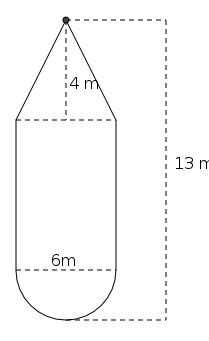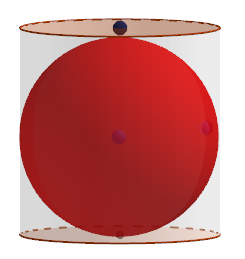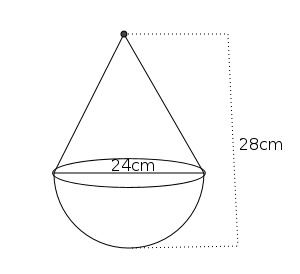Question Pool Solids

# Solids - SAMAGRA Question Pool & Answers | Class 10 English Medium

Kerala Syllabus SAMAGRA SCERT SAMAGRA Question Pool for Class 10 English Medium Maths SolidsQn 1.

The base radius and height of cylinder are 10 cm, 12 cm respectively.

a). Find its volume

b). Find the volume of the largest cone that can be carved out from this cylinder.

Get Free Study Materials + 1 Week Free Trial of BrainsPrep Class 10 English Medium Tuition

Qn 2.

The radii of a cone, a hemisphere, a cylinder and a sphere are r units. The heights of the cone and cylinder are equal to their radii.Prove that the volumes of  the cone , the hemisphere , the cylinder and the  sphere are in arithmetic sequence .

Get Free Study Materials + 1 Week Free Trial of BrainsPrep Class 10 English Medium Tuition

Qn 3.

What is the surface area of a hemisphere of radius 9 cm?

Get Free Study Materials + 1 Week Free Trial of BrainsPrep Class 10 English Medium Tuition

Qn 4.The Shape of a boiler is a hemisphere attached to one end and a cone attached to the other end of a cylinder as in the figure. Diameter of the cylinder is 6 m and height of the cone is 4m and total height is 13m.

a). What is the height of the cylinder?

b). How many litres of water can the boiler contain?

Get Free Study Materials + 1 Week Free Trial of BrainsPrep Class 10 English Medium Tuition

Qn 5.

The base perimeter of a square pyramid is 40 cm and the area of one of its lateral face is 65 sq. Cm

a).Find its base edge?

b).Find its slant height ?

c)Find its volume.

Get Free Study Materials + 1 Week Free Trial of BrainsPrep Class 10 English Medium Tuition

Qn 6.

The base edge and height of a square prism are 10 cm and 12 cm respectively.

a). Find its volume

b). Find the volume of the square pyramid of maximum size that can be carved out from this prism.

Get Free Study Materials + 1 Week Free Trial of BrainsPrep Class 10 English Medium Tuition

Qn 7.

A cone of maximum size is carved out from a hemisphere of radius 10 cm.

a). What is the base radius of the cone ?.

b). Find the slant height

c). Find the curved surface area of the cone?

Get Free Study Materials + 1 Week Free Trial of BrainsPrep Class 10 English Medium Tuition

Qn 8.

A sector of radius 18cm and central angle2400 is rolled to form a cone

a).What is the slant height of the cone?

b).What is the base radius of the cone ?

c). Find the curved surface area of the cone?

Get Free Study Materials + 1 Week Free Trial of BrainsPrep Class 10 English Medium Tuition

Qn 9.

A sphere of maximum size is carved out from a cube of side 12 cm.

a). Find radius of the sphere?

b).Find the volume of the sphere?

Get Free Study Materials + 1 Week Free Trial of BrainsPrep Class 10 English Medium Tuition

Qn 10.The diameter and height of a wooden cylinder are equal.

A sphere of maximum size is carved out from this. Height of the cylinder is 10 cm. Then,

a).Find the radius of the sphere

b).Find the ratio of the surface areas of the cylinder and sphere

Get Free Study Materials + 1 Week Free Trial of BrainsPrep Class 10 English Medium Tuition

Qn 11.

A sector is cut out from a circle drawn on a paper. A cone of radius 9 cm and height 12 cm is made using the sector

a) What was the radius of the circle?

b) What was the central angle of the sector?

Slant height = 15 cm

Radius of the circle = 15 cm

Central angle

Get Free Study Materials + 1 Week Free Trial of BrainsPrep Class 10 English Medium Tuition

Qn 12.

How many solid cones with height 5 cm and radius 6 cm can be made by recasting a solid metal sphere of radius 15 cm?

Get Free Study Materials + 1 Week Free Trial of BrainsPrep Class 10 English Medium Tuition

Qn 13.

The radius of a cone and a sphere are 10 cm each. If their volumes are equal what will be the height of the cone?

Get Free Study Materials + 1 Week Free Trial of BrainsPrep Class 10 English Medium Tuition

Qn 14.

The lateral faces of a square pyramid are equilateral triangles. If the base edge of the pyramid is 18 cm

a) What will be the height of the pyramid?

b) Find the volume of the pyramid

Get Free Study Materials + 1 Week Free Trial of BrainsPrep Class 10 English Medium Tuition

Qn 15.

8 spheres , each of volume 36π cm3 are melt and recast in to a large sphere

a). What is the volume of the large sphere?

b). What will be the radius of the large sphere?

volume of the large sphere  = 36 π x 8 = 288π cm3.

4 /3 πr3 = 288 π

Get Free Study Materials + 1 Week Free Trial of BrainsPrep Class 10 English Medium Tuition

Qn 16.

The shape of a toy is as shown in the figurea) What is the height of the cone?.

b)Find the total surface area of the toy ?.

Height of the cone = 16

Get Free Study Materials + 1 Week Free Trial of BrainsPrep Class 10 English Medium Tuition

Qn 17.

How many spheres of radius 3 cm can be made by melting and recasting a metal cone of radius 12 cm and height 15 cm?.

Volume of the cone =  720π cm3

Volume

Get Free Study Materials + 1 Week Free Trial of BrainsPrep Class 10 English Medium Tuition

Qn 18.

Two square pyramids have equal volume. Base edge and height of the first pyramid are 12 cm and 20 cm respectively. If base edge of the second pyramid is 8 cm, what is its height ?

Get Free Study Materials + 1 Week Free Trial of BrainsPrep Class 10 English Medium Tuition

Qn 19.

Base area of a square pyramid is 324 sq.cm. And its height is 12 cm. Find its base  edge, slant height and surface area.

Get Free Study Materials + 1 Week Free Trial of BrainsPrep Class 10 English Medium Tuition